# Linear Equations

Parent topic:
Equations
•### Graph the Line

Activity

Steve Phelps

•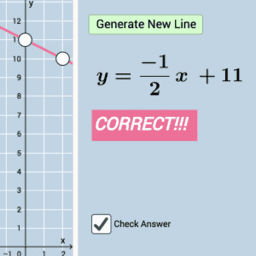### Graphing Linear Equations: Formative Assessment Items

Book

Tim Brzezinski

•### Quiz: Graphing Linear Equations (V3)

Activity

Tim Brzezinski

•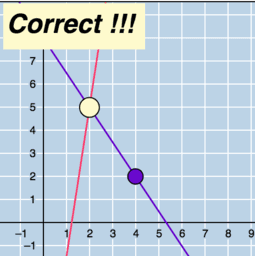### Solving Linear Systems by Graphing: Quiz (V1)

Activity

Tim Brzezinski

•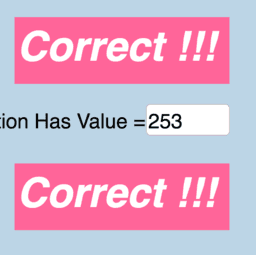### Linear Equation Generator

Activity

Tim Brzezinski

•### Quiz: Graphing Linear Equations (V1)

Activity

Tim Brzezinski

•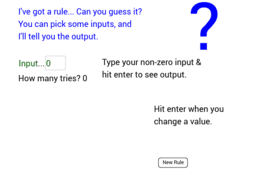### Guess My Rule (2013)

Activity

John Golden

•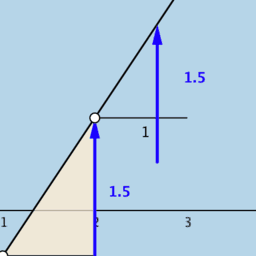### Equation of a Line: Dynamic Illustrator

Activity

Tim Brzezinski

•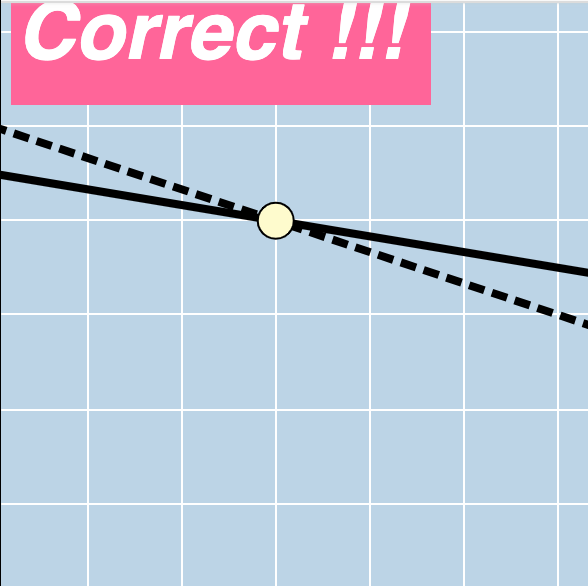### Solving Linear Systems by Graphing: Quiz (V2)

Activity

Tim Brzezinski

•### Writing the Equation of a Line (I-VA)

Activity

Tim Brzezinski

•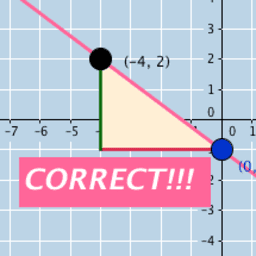### Quiz: Graphing Linear Equations (V2)

Activity

Tim Brzezinski

•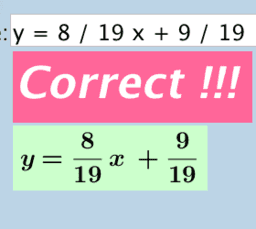### Standard Form to Slope-Intercept Form (Rewriting Linear Equations)

Activity

Tim Brzezinski

•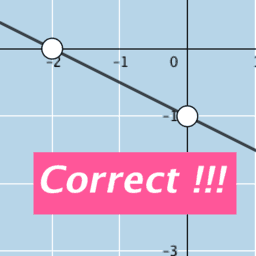### Graphing Linear Equations Written in Standard Form: Quiz (V1)

Activity

Tim Brzezinski

•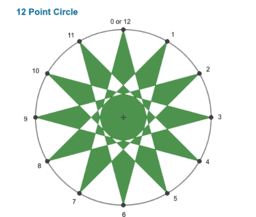### Apps for Formative Assessment Grade 10

Book

Lee W Fisher

•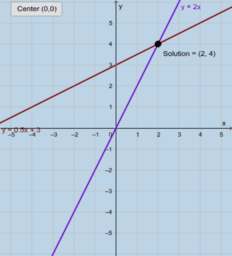### Solving Linear Systems Graphically

Activity

Tim Brzezinski

•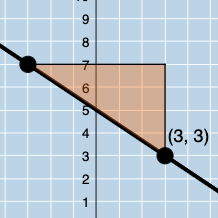### Writing the Equation of a Line Given a Slope & Point (V1)

Activity

Tim Brzezinski

•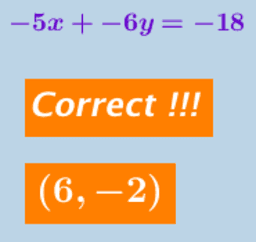### Solving Linear Systems Algebraically: Quiz (V2)

Activity

Tim Brzezinski

•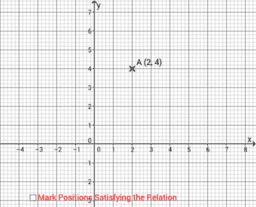### Graphing Linear Equations in 2 Unknowns

Activity

Anthony OR 柯志明

•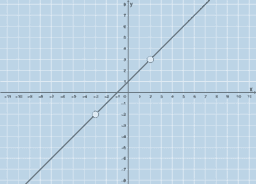### Working with Linear Equations Written in Standard Form: Quiz (V2-A)

Activity

Tim Brzezinski

•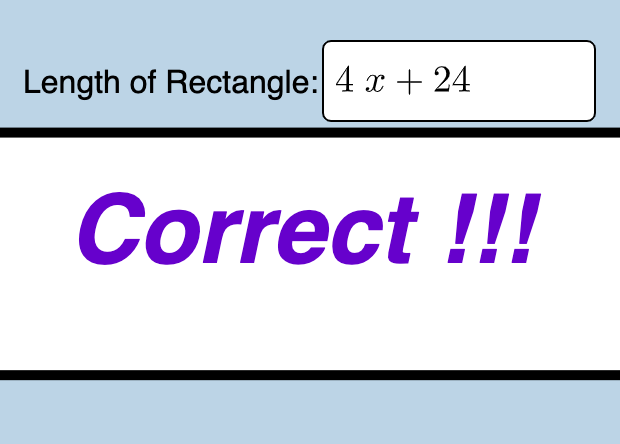### Application Problems: Solving Linear Equations (2)

Activity

Tim Brzezinski

•### Quiz: Modeling with Linear Functions

Activity

Tim Brzezinski

•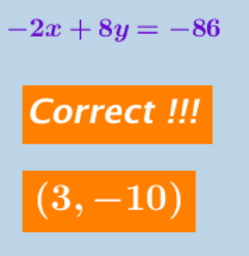### Solving Linear Systems Algebraically: Quiz (V1)

Activity

Tim Brzezinski

•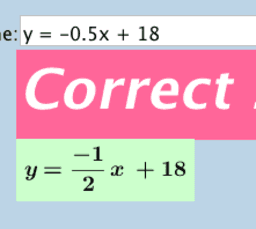### Writing the Equation of a Line Given 2 Points (V1)

Activity

Tim Brzezinski

•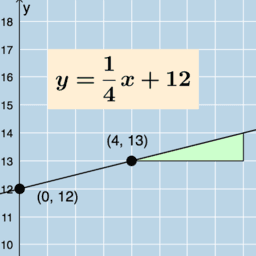### Relating SLOPE and Y-INTERCEPT to the REAL WORLD

Activity

Tim Brzezinski